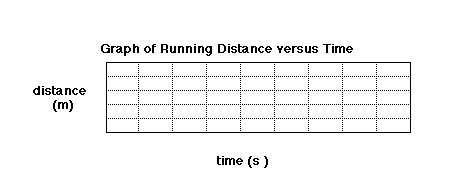## Saturday, 18 February 2006

### Fisika SMA

Pengalaman Belajar Fisika di SMAN BI 1 Banjar

## Lesson Plan: Graphing Data from a Spreadsheet

SUBJECT: Technology
TOPIC: Presenting Data
DESCRIPTION: A set of problems dealing with graphing data.
CONTRIBUTED BY: Carol Hodanbosi
EDITED BY: Jonathan G. Fairman - August 1996

Purpose:
To graph real data to input into a computer spreadsheet
Objective:
To illustrate a distance versus time graph using student data from running various distances.
Materials:
• computer spreadsheet with data from previous lab activity
• computer graphing utility
Procedure:
1. Each student will be graphing the average of their classes running speed data, putting distance (from 0 meters to 20 meters on the vertical or Y axis, and time on the X axis ( in seconds), in 0.1 second intervals.
2. Plot the values for your class, using the average time for the 5, 10, and 15 meter distances.
3. Plot a fourth point using a zero distance, and estimating what the time would be for that distance.
4. Draw a straight, best-fit line through the data points.
5. Using the points for the 15 meter time and the zero point as endpoints, calculate the slope of the line segment.
6. What does the slope represent?
7. Can you predict the slope of the line if the distance were extended to a much greater value, such as 10,000 meters (10k), or 40,000 meters?Sumber:
NASA